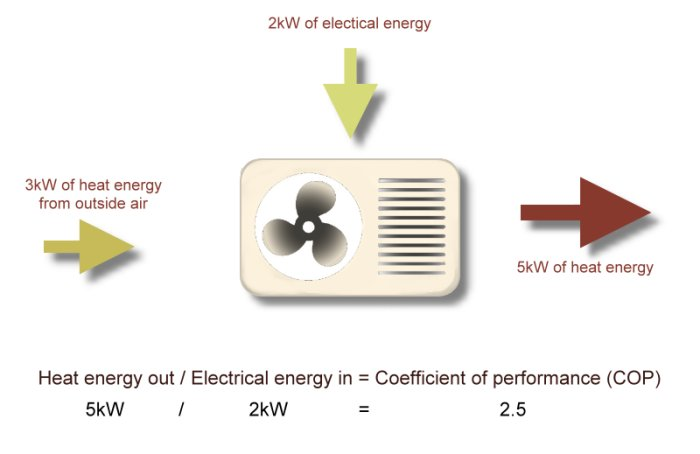What are you looking for?# COP and SPF for Heat Pumps Explained

Heat pumps are powered by electricity but unlike conventional electrical heating, a heat pump is highly efficient. If you are looking into heat pumps, you’ll have come across the terms COP and SPF.

Coefficient of Performance (COP) of Heat Pumps

COP (Coefficient of Performance) is a measure of efficiency. The efficiency of any machine or system can be calculated as the ratio of amount of work done by the machine to the amount of work given to the machine. In the case of a heat pump, its efficiency is the ratio of useful heat energy produced to electrical energy consumption. A COP of 2.5 means that the heat pump supplies 2.5 times as much heat energy to the system as it consumes in electrical energy.

The first law of thermodynamics tells us that energy can neither be created nor destroyed and this makes it hard to understand how a machine can generate more energy than it consumes. You’ll even find the odd Internet forum where someone states this and claims heat pump efficiency of more than one is “scientifically” impossible. The answer here is that COP measures how efficient a heat pump is at turning electrical energy into usable heat. It doesn’t do this by creating energy or being more than 100% efficient. It does it by using heat energy already present in the outside air, ground or water.

In technical formula, COP is written as Q/W, where Q is the heat generated and W is the power used. Assuming 5KW is the heat output by the heat pump. And that it generates this by using 2kW of electrical energy and 3kW of energy from outside air. Then the COP of the heat pump will be 2.5.In case of an air source heat pump, COP will be higher when outside air temperature is higher, as less electrical energy input will be needed to generate a given heat output. The same is true for a ground source heat pump but ground temperature will vary less and won’t drop as low, as quickly, in winter. The COP of a heat pump if mentioned on the machine itself will tell us how many units of heat/cold can be produced per unit of electricity under test conditions.

Seasonal Performance Factor (SPF) of Heat Pumps

Where COP gives us efficiency of a heat pump at any given time, SPF gives us the same but for annual performance of the heat pump. Air source heat pumps use heat energy from the outside air and ground source heat pumps use heat energy from the ground. The heat pump needs to raise the temperature of this outside source to that needed by the heating system. Outside temperature is obviously lower in winter and higher in summer and this affects the amount of work the heat pump needs to do. At lower outside temperatures the heat pump will need more electrical energy to produce a given heat output, meaning that the system is less efficient. As a result, the COP will be different through the seasons and SPF encompasses this by considering the annual performance of the system.

The SPF of a heat pump is the ratio of annual heat generated to the annual electricity consumed for the operation of the heat pump.

As a formula this is calculated as:

SPF = Total heat output per annum / Total electricity consumed per annum

SPF tells how efficient a heat pump is on average.